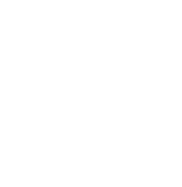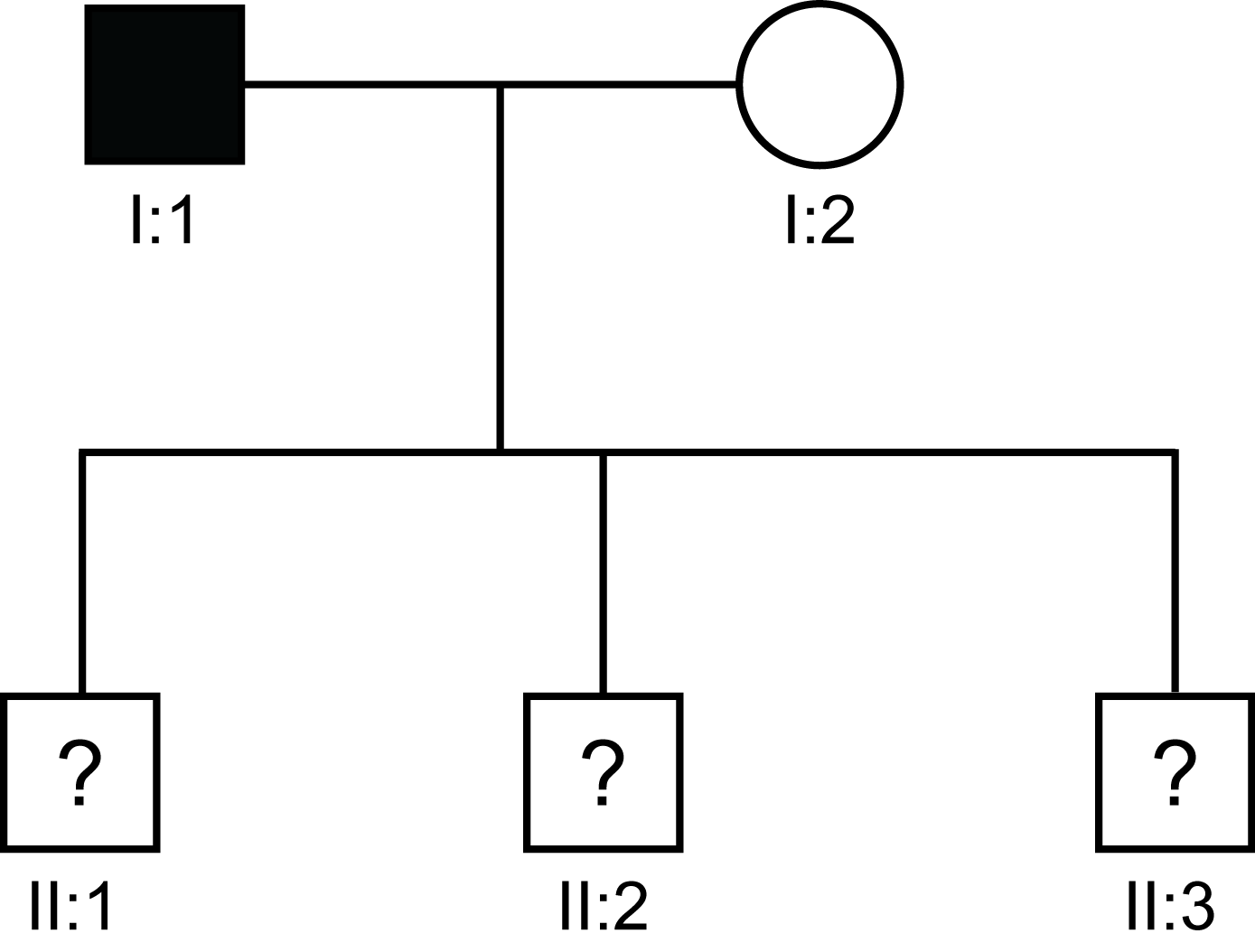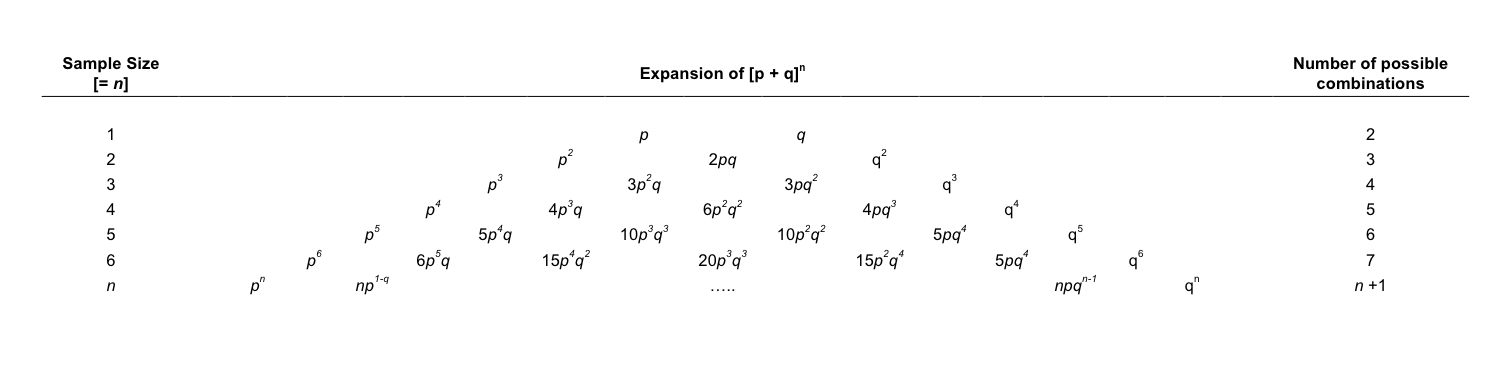:

# Practical-Haemostasis.com## A Practical Guide to Haemostasis

Genetics:
Pascal's Triangle and Risk Calculations

### Introduction

Pascal's Triangle, based upon the French Mathematician Blaise Pascal, is used in genetic counselling to calculate the probability of obtaining a particular number or distribution of events of one kind knowing the probability of each event occurring independently. This is a simpler approach to the use of the Binomial Distribution.

### Principles: Pascal's Triangle

The simplest way to look at the role of Pascal's Triangle is to study a family with an autosomally inherited disorder.

Consider the following scenario:
A 45-year-old man is diagnosed with Type 1 Antithrombin deficiency [AT:Act 45 U/dL] and is concerned that he may have passed it onto his three children. His partner has normal functional Antithrombin levels. He consults you and asks what is the risk that his children will have Type 1 Antithrombin deficiency.
Antithrombin deficiency is inherited as an autosomal dominant disorder.

The Family Pedigree is shown below:Squares = males
Circles = females
Solid square - male with Type 1 Antithrombin Deficiency
? - Phenotype/Genotype unclear

He consults you and asks what are the risks that:
1. All 3 of his children [II:1, II:2 and II:3] will have Type 1 Antithrombin deficiency.
2. All 3 of his children [II:1, II:2 and II:3] will not have Type 1 Antithrombin deficiency?
3. 1 of his children will have Type 1 Antithrombin deficiency?
4. 2 of his children will have Type 1 Antithrombin deficiency?

We can derive the answers for these questions using Pascal's Triangle - derived by expanding (p +q)n. This is shown diagrammatically below:p = the probability of an event not occurring - in this case not inheriting the disorder
q = the probability of an event occurring  - in this case inheriting the disorder
n = the total number of disorders - in this case the number of potential or actual offspring

So - if we look at the family with 3 possible children [n = 3] and if read along the Triangle, we see that for all 3 of the children to have Type I Antithrombin deficiency the probability is [q]3 where q = 1/2 and so [1/2]3 = 1/8.
Antithrombin deficiency is an autosomally inherited disorder and the risk of inheriting a mutant allele from a parent is 1/2 and of not inheriting it, is also 1/2.   So the probability that all 3 children will be normal [p]3 is 1/8 and similarly the probability that all 3 children will have Type 1 Antithrombin deficiency, is [q]3 which is also 1/8.

To establish if only 1 of the children will be affected and the other two normal then again we read along from n=3 to where we see 3pq2 and so 3pq2 = 3 x 1/2 x [1/2]2 = 3 x 1/2 x 1/4 = 3/8. So the risk that they will have one affected child and two normal children is 3/8.
Remember p is the probability of an event not occurring - in this case not inheriting the disorder and q is the probability of an event occurring - in this case inheriting the disorder.

Finally the risk that they will have two affected children and one normal child is 3p2q = 3 x [1/2]2 x 1/2 = 3/8.
The probabilities of all the possibilities must add up to 1 i.e. [p]3+ [q]3 + 3pq2 + 3p2q  [1/8 + 1/8 + 3/8 + 3/8] = 1.

If they had a further child, then the Sample SIze = 4 but the method of calculating the various risks is the same - just read along from n=4 to derive the formulae for calculating the risks. The principle is the same for all autosomally inherited disorders.